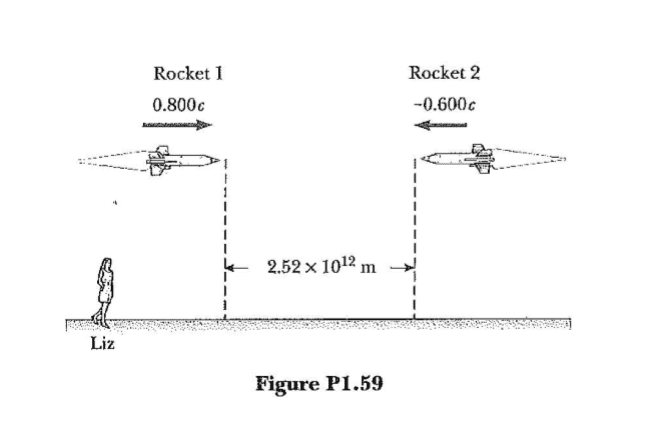# Should I use time dilation or length contraction?

• Joao Victor Dantas
According to rocket 1, how long before they collide? According to rocket 1, it will take it 88.9 minutes to reach the point of collision.f

#### Joao Victor Dantas

1. Homework Statement [/B]

This is a problem that was in my Physics HW.

Two powerless rockets are on a collision course. The rockets are moving with speeds of 0.800c and 0.600c and are initially ## 2.52 × 10^{12} ## m apart as measured by Liz, an Earth
observer, as shown in Figure P1.59. Both rockets are 50.0 m in length as measured by
Liz. (a) What are their respective proper lengths? (b) What is the length of each rocket as
measured by an observer in the other rocket? (c) According to Liz, how long before the
rockets collide? (d) According to rocket 1, how long before they collide? (e) According
to rocket 2, how long before they collide? (f) If both rocket crews are capable of total
evacuation within 90 minutes (their own time), will there be any casualties?

My doubt is on letters (d) and (e). I don't know if I am supposed to apply the time Lorentz transformation using the value obtained in (c) or if I should calculate this time based on the speed each rocket sees the other approaching and the distance using length contraction. I found two answers on the internet.## Homework Equations

## L = L_{0}\sqrt {1 - \frac {v^2} {c^2}} ##
## \Delta t' = \frac 1 {\sqrt {1 - \frac {v^2} {c^2}}} \Delta t ##
## V' = \frac {u - V_x} {1 - \frac {uV_x} {c^2}} ##

## The Attempt at a Solution

By using the mentioned equations, I obtained that (a) ## L_1 = 83.3 m ## and ## L_2 = 62.5 m ## . (b) ## L_1 = 27.0 m ## in the frame of rocket 2 and ## L_2 = 21.0 m ## in the frame of rocket 1. (c) ## \frac {\Delta S} {v_1 + v_2} = 6000 sec = 100 min ## .

When it comes to letter (d) that something goes wrong. my first approach to it was to use the length contraction observed by 1 and divide it by the speed 1 sees 2 approaching. ## L = L_{0}\sqrt {1 - \frac {v^2} {c^2}} = 2.52 \times 10^{12} \times 0.6 = 1.512 \times 10^{12} ## and ## V' = \frac {u - V_x} {1 - \frac {uV_x} {c^2}} = \frac { 0.8c - ( - 0.6c)} {1 - \frac { (- 0.48c^2)} { c^2 }} = 0.945c ## . Dividing these results we have ## \frac {L} {V'} = 5,333 sec = 88.9 min ## . Although, using ## \Delta t' = \frac 1 {\sqrt {1 - \frac {v^2} {c^2}}} \Delta t ## , where t' is Liz's time of 100 min, we obtain ## 100 min = 1.6666 \times \Delta t ## and ## \Delta t = 60 min ##. This same problem happens when I try to solve (e), and I've taken a look at several solutions on the internet, being half of them solved in the first way, and half in the second. Shouldn't these results agree? If not, why?

#### Attachments

Last edited by a moderator:
As with most relativity problems, the difficulty is with the relativity of simultaneity. According to Liz on Earth, the start event for both Liz and L1 is simultaneous. The end event is a collision and is naturally simultaneous for all parties involved. According to L1, the start event for L1 is not simultaneous with the start event for Liz.

•Joao Victor Dantas
This makes sense. So 88.9 min would be the time it takes for the observer in rocket 1 to get to the point of collision from the start of HIS measurement and 60 min would be the time is takes for this observer from the start of Liz's measurement, correct?

Can you draw a position-vs-time graph of the problem?

This makes sense. So 88.9 min would be the time it takes for the observer in rocket 1 to get to the point of collision from the start of HIS measurement and 60 min would be the time is takes for this observer from the start of Liz's measurement, correct?
I am not sure that I understand your phrasing here. Consider that L1 has a stopwatch. He starts it at some point. And we are asked for its reading at the event of the collision.

At what event does L1 start his stopwatch? I would suggest having him start it at the event that Liz considers to be simultaneous with the scenario start.

Maybe it would help to resolve the issue if you calculated the "contracted" distance that
each ship travels and then the time to traverse this distance.
Use the 100 min. that you calculated as measured by Liz.